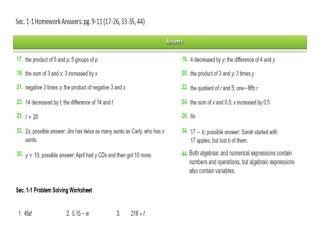DownloadDownload PresentationTo evaluate an expression is to find its value. To evaluate an algebraic expression, substitute

# To evaluate an expression is to find its value. To evaluate an algebraic expression, substitute

Download Presentation## To evaluate an expression is to find its value. To evaluate an algebraic expression, substitute

- - - - - - - - - - - - - - - - - - - - - - - - - - - E N D - - - - - - - - - - - - - - - - - - - - - - - - - - -
##### Presentation Transcript

1. Vocabulary To evaluatean expression is to find its value. To evaluate an algebraic expression, substitute numbers for the variables in the expression and then simplify the expression.

2. Example 3: Evaluating Algebraic Expressions Evaluate each expression for a = 4, b =7, and c = 2. A. b – c 7 – 2 Substitute 7 for b and 2 for c. = 5 Simplify. B. ac Substitute 4 for a and 2 for c. 4 ·2 = 8 Simplify.

3. Check It Out! Example 3 Evaluate each expression for m = 3, n = 2, and p = 9. a.mn mn = 3 · 2 Substitute 3 for m and 2 for n. = 6 Simplify. b. p – n Substitute 9 for p and 2 for n. p – n = 9 – 2 = 7 Simplify. c. p ÷ m p ÷ m = 9 ÷ 3 Substitute 9 for p and 3 for m. = 3 Simplify.

4. Example 4A: Recycling Application Approximately eighty-five 20-ounce plastic bottles must be recycled to produce the fiberfill for a sleeping bag. Write an expression for the number of bottles needed to make s sleeping bags. The expression 85s models the number of bottles to make s sleeping bags.

5. Example 4B: Recycling Application Continued Approximately eighty-five 20-ounce plastic bottles must be recycled to produce the fiberfill for a sleeping bag. Find the number of bottles needed to make 20, 50, and 325 sleeping bags. Evaluate 85s for s = 20, 50, and 325. To make 20 sleeping bags, 1700 bottles are needed. 85(20) = 1700 To make 50 sleeping bags, 4250 bottles are needed. 85(50) = 4250 To make 325 sleeping bags, 27,625 bottles are needed. 85(325) = 27,625

6. Writing Math A replacement set is a set of numbers that can be substituted for a variable. The replacement set in Example 4 is (20, 50, and 325).

7. Check It Out! Example 4a To make one sweater, 63 twenty ounce plastic drink bottles must be recycled. Write an expression for the number of bottles needed to make s sweaters. The expression 63s models the number of bottles to make s sweaters.

8. Check It Out! Example 4b Continued To make one sweater, 63 twenty ounce plastic drink bottles must be recycled. Find the number of bottles needed to make 12, 25 and 50 sweaters. Evaluate 63s for s = 12, 25, and 50. To make 12 sweaters, 756 bottles are needed. 63(12) = 756 To make 25 sweaters, 1575 bottles are needed. 63(25) = 1575 To make 50 sweaters, 3150 bottles are needed. 63(50) = 3150

9. Homework Assignment Pg. 9-11 (27-31, 36, 39-41, 45-47) & Sec. 1-1 Problem Solving Wksht (4-7)